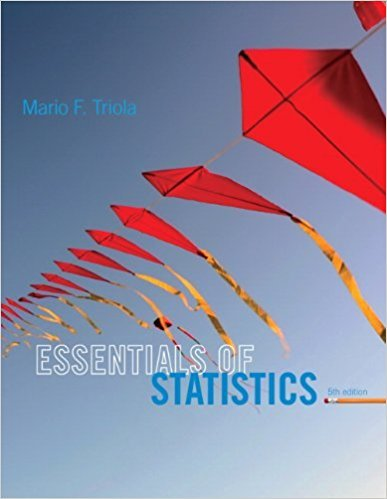×
×

# Solutions for Chapter 11.2: Goodness-of-Fit## Full solutions for Essentials of Statistics | 5th Edition

ISBN: 9780321924599Solutions for Chapter 11.2: Goodness-of-Fit

Solutions for Chapter 11.2
4 5 0 275 Reviews
13
1
##### ISBN: 9780321924599

This expansive textbook survival guide covers the following chapters and their solutions. This textbook survival guide was created for the textbook: Essentials of Statistics, edition: 5. Chapter 11.2: Goodness-of-Fit includes 25 full step-by-step solutions. Essentials of Statistics was written by and is associated to the ISBN: 9780321924599. Since 25 problems in chapter 11.2: Goodness-of-Fit have been answered, more than 15556 students have viewed full step-by-step solutions from this chapter.

Key Statistics Terms and definitions covered in this textbook

A formula used to determine the probability of the union of two (or more) events from the probabilities of the events and their intersection(s).

• Arithmetic mean

The arithmetic mean of a set of numbers x1 , x2 ,…, xn is their sum divided by the number of observations, or ( / )1 1 n xi t n ? = . The arithmetic mean is usually denoted by x , and is often called the average

• Attribute

A qualitative characteristic of an item or unit, usually arising in quality control. For example, classifying production units as defective or nondefective results in attributes data.

• Axioms of probability

A set of rules that probabilities deined on a sample space must follow. See Probability

• Block

In experimental design, a group of experimental units or material that is relatively homogeneous. The purpose of dividing experimental units into blocks is to produce an experimental design wherein variability within blocks is smaller than variability between blocks. This allows the factors of interest to be compared in an environment that has less variability than in an unblocked experiment.

• Categorical data

Data consisting of counts or observations that can be classiied into categories. The categories may be descriptive.

• Completely randomized design (or experiment)

A type of experimental design in which the treatments or design factors are assigned to the experimental units in a random manner. In designed experiments, a completely randomized design results from running all of the treatment combinations in random order.

• Conditional probability mass function

The probability mass function of the conditional probability distribution of a discrete random variable.

• Control chart

A graphical display used to monitor a process. It usually consists of a horizontal center line corresponding to the in-control value of the parameter that is being monitored and lower and upper control limits. The control limits are determined by statistical criteria and are not arbitrary, nor are they related to speciication limits. If sample points fall within the control limits, the process is said to be in-control, or free from assignable causes. Points beyond the control limits indicate an out-of-control process; that is, assignable causes are likely present. This signals the need to ind and remove the assignable causes.

• Correlation coeficient

A dimensionless measure of the linear association between two variables, usually lying in the interval from ?1 to +1, with zero indicating the absence of correlation (but not necessarily the independence of the two variables).

• Covariance

A measure of association between two random variables obtained as the expected value of the product of the two random variables around their means; that is, Cov(X Y, ) [( )( )] =? ? E X Y ? ? X Y .

• Covariance matrix

A square matrix that contains the variances and covariances among a set of random variables, say, X1 , X X 2 k , , … . The main diagonal elements of the matrix are the variances of the random variables and the off-diagonal elements are the covariances between Xi and Xj . Also called the variance-covariance matrix. When the random variables are standardized to have unit variances, the covariance matrix becomes the correlation matrix.

• Decision interval

A parameter in a tabular CUSUM algorithm that is determined from a trade-off between false alarms and the detection of assignable causes.

• Deining relation

A subset of effects in a fractional factorial design that deine the aliases in the design.

• Deming’s 14 points.

A management philosophy promoted by W. Edwards Deming that emphasizes the importance of change and quality

• Dispersion

The amount of variability exhibited by data

• Distribution free method(s)

Any method of inference (hypothesis testing or conidence interval construction) that does not depend on the form of the underlying distribution of the observations. Sometimes called nonparametric method(s).

• Error mean square

The error sum of squares divided by its number of degrees of freedom.

• Error of estimation

The difference between an estimated value and the true value.

• Gamma random variable

A random variable that generalizes an Erlang random variable to noninteger values of the parameter r

×